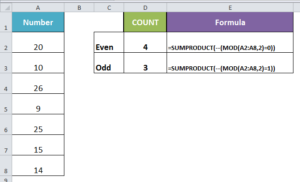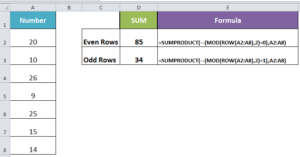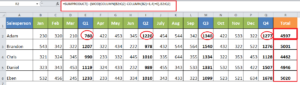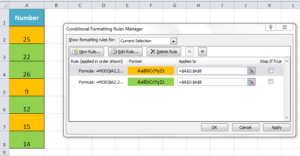Get instant live expert help with Excel or Google Sheets“My Excelchat expert helped me in less than 20 minutes, saving me what would have been 5 hours of work!”

#### Post your problem and you’ll get expert help in seconds.

Your message must be at least 40 characters
Our professional experts are available now. Your privacy is guaranteed.

# How to Use the MOD Function in Excel

In Excel, sometimes you need to deal with some complex tasks like to sum or highlight cells that have even or odd numbers, sum every Nth row or column in a range, validate cells to enter numbers with even or odd or without a fraction, and many more. To solve these tricky problems you can use the MOD function.

## The Excel MOD Function

Though Excel MOD function is basically designed to get the division remainder, it is far more useful to solve many complex problems like above while working with data.  The MOD function returns the remainder after a number is divided by a divisor. In Excel, it is categorized as a Math & Trig Function and it returns a numeric value.

### The MOD formula

The syntax of the MOD function is:

`MOD( number, divisor )`

Where,

Number: A numeric value whose remainder is needed.

Divisor: The number used to divide the number parameter.

• If the divisor is 0, then it will return the #DIV/0 error because a number cannot be divided by 0.
• If any of two arguments is text value then it will return #VALUE error because it handles only numeric values.

For example, if you divide the numbers by divisors, then it will return remainder values by using the MOD function as follows;

It is rare that the MOD function is used to get the only remainder of division in Excel. The MOD function is quite often used in formulas to make calculations based on the remainder of the divisor in Excel. In this article, you will learn how to use the MOD function in Excel to solve various advanced problems to reach at solutions easily.

### Count cells that contain odd or even numbers

Excel does not have any built-in function to perform such a count, but the MOD function can do this in the formula using the MOD and SUMPRODUCT functions. If a value is divided by 2 and the remainder is 0, then it is an even value, but if the remainder is 1 then the value is odd. Using the MOD function you can perform this check on which cell has even or odd values in a selected range of cells, and the SUMPRODUCT function counts the total cells where this condition is TRUE.

Suppose you have numbers in a range of cells A2:A8 and you need to count cell that have Even or Odd numbers using the following formulas;

Count cells with Even numbers:

`=SUMPRODUCT(--(MOD(A2:A8,2)=0))`

Count cells containing Odd numbers:

`=SUMPRODUCT(--(MOD(A2:A8,2)=1))`

Here, MOD function divides each cell’s value in a range by 2, and if remainder in the result is 0 then it is even valued and if the remainder is 1 then it is an odd number. Excel returns a series of TRUE and FALSE where these logical tests are met or not in the above formulas. Double dashes (–) outside the MOD function converts series of TRUEs and FALSEs into 1s and 0s and SUMPRODUCT function sums this series of 1s and 0s to give you the total count of cells.### Sum every other row or column

Based on even and odd numbers logic, you can sum every other row or column in a selected range of cells using MOD, ROW or COLUMN and SUMPRODUCT functions. In this case, you can sum every even or odd row or column number in the selected range of cells.

Suppose you have a range of cells with a number and you want to sum every even or odd row then formulas will be as follows;

Sum every Even row:

`=SUMPRODUCT(--(MOD(ROW(A2:A8),2)=0),A2:A8)`

Sum every Odd row:

`=SUMPRODUCT(--(MOD(ROW(A2:A8),2)=1),A2:A8)`

Here, MOD function will perform check where row number in the selected range is even or odd, and SUMPRODUCT function will sum the values of even or odd row numbers in the above formulas.### Sum every Nth row or column

There is no built-in function in Excel to sum values of every 2nd, 3rd or 4th etc. row or column in a range. But, using the MOD function with the combination of ROW or COLUMN and SUMPRODUCT functions in formulas you can perform such calculations easily by following this generic formulas syntax;

`SUMPRODUCT(--(MOD(ROW(range)-ROW(first_cell)+1,n)=0),range)`

OR

`SUMPRODUCT(--(MOD(COLUMN(range)-COLUMN(first_cell)+1,n)=0),range)`

Where n is the Nth row or column to be summed in provided range

Suppose you have monthly and quarterly sales figures for salespersons and you want to sum the sales of all quarters of each salesperson. So using the MOD function you can perform this calculation as per the following formula.

`=SUMPRODUCT(--(MOD(COLUMN(B2:Q2)-COLUMN(B2)+1,4)=0),B2:Q2)`

In this example sales figures are provided column wise and quarterly sales figure of every salesperson is available in 4th column of the selected range for each salesperson. So, you need to sum every 4th column figure in each range.### Highlight odd and even numbers

Using conditional formatting custom formula you can conditionally highlight odd and even number in a range by using MOD function.

Select the range of cells that you need to conditionally highlight. Go to Home tab > Style section> click on Conditional Formatting > New Rule > Use formula to determine which cells to format

You need to add the following two formulas in Conditional Formatting Rule Manager dialog box for even and odd numbers and select separate formatting style for each.

The formula for cells with even numbers:

`=MOD(\$A2,2)=0`

Format > Fill = Green

The formula for cells with odd numbers:

`=MOD(\$A2,2)=1`

Format > Fill = GoldStill need some help with Excel formatting or have other questions about Excel? Connect with a live Excel expert here for some 1 on 1 help. Your first session is always free.

### Did this post not answer your question? Get a solution from connecting with the expert.Another blog reader asked this question today on Excelchat:
Solution examplesI have a list or people who are ranked in numbers from 3 to 6 I need to recognize the contents of each multiple cells and create an equivalent letter. for that value into another column. e.g. Cell E1 = 3 to show in new cell that row (H1) the letter "C" I have multiple rows with different values in column "E" Can this be done?
Solved by I. J. in 30 minsI need to create a formula that fills finds the Sheet (col A) and then the Component (col B) and then adds the value I put in Col H into a cell in the corresponding cell of column of the found sheet.
Solved by Z. S. in 60 minsI need to count number of dates from a timestamped date in column from the completed date column to show the number of days. I have not successfully been able to do this. I am a novice Excel user. Can you help?
Solved by O. B. in 15 minsMy VLOOKUP formula won't work when value the "lookup cell" is derived from a formula. If I manually transfer the formula derived value to a different cell, then VLOOKUP formula works fine. What's going on?
Solved by Z. J. in 40 minsMY QUESTION IS ABOUT: Fixing Formulas DESCRIPTION: Search thru Column H and Column I, and IF the cell CONTAINs STRING A, STRING B, OR STRING C, return VALUE 1 OR if cell CONTAINs String D, String E, OR STRING F, return VALUE 2, OR if cell CONTAINs String G, OR String H, return VALUE 3 Otherwise, return UNKNOWN.
Solved by M. H. in 60 mins## Subscribe to Excelchat.coAnother blog reader asked this question today on Excelchat: